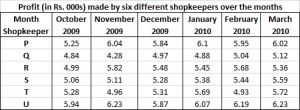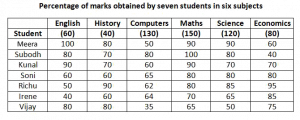# IBPS Clerk Data Interpretation Questions

0
3456

Every year 5 to 10 questions will be asked from Data interpretation topic. This questions lies form easy to moderately difficult. If you are good at approximations and calculations then you could solve this questions in very less time compared to others. Data interpretation mostly appear as sets of pie charts, bar graphs, line graphs, tables, case-lets and other miscellaneous forms. So, here we are providing one set of solved questions and answers on Data Interpretation for IBPS Clerk. Try this solved DI problems of Bank clerk on your before viewing the given solution.

IBPS Clerk Data Interpretation Questions:

Practice IBPS Clerk Computer Awareness online tests and download all GK ebooks for Banking exams

Instructions (1 – 5) :

Direction: Study the table carefully to answer the questions that follow.Question 1:

What is the respective ratio between the profit earned by shopkeeper U in the months February 2010 and March – 2010 together to that earned by shopkeeper Q in the same months ?

a) 637: 512
b) 621 : 508
c) 512: 637
d) 508: 621
e) None of these

Question 2:

What is the percent increase in profit of shopkeeper S in the month of December – 2009 over the previous month ? (rounded off to two digits after decimal)

a) 3.15
b) 2.67
c) 2.18
d) 3.33
e) None of these

Questions 3:

Which shopkeeper’s profit kept increasing continuously over the given months ?

a) R
b) Q
c) T
d) U
e) None of these

Question 4:

What is the difference in profit earned by shopkeeper T in January – 2010 from the previous month ?

a) Rs. 640/-
b) Rs. 420/-
c) Rs. 380/-
d) Rs. 760/-
e) None of these

Question 5:

What was the average profit earned by shopkeeper R in the months of October 2009 and November 2009 together ?

a) 5405
b) 5040
c) 4825
d) 4950
e) None of these

Instructions (6 – 10) :

Study the following table to answer the questions:Question 6:

What are the total marks obtained by Meera in all the subjects ?

a) 448
b) 580
c) 470
d) 74.67
e) None of these

Question 7:

What are the average marks obtained by these seven students in History ?

a) 72.86
b) 27.32
c) 24.86
d) 29.14
e) None of these

Question 8:

How many students have got 60% or more marks in all the subjects ?

a) One
b) Two
c) Three
d) None
e) None of these

Question 9:

What is the overall percentage of Kunal ?

a) 64
b) 65
c) 75
d) 64.24
e) None of these

Question 10:

In which subjects is the overall percentage the best ?

a) Maths
b) Economics
c) History
d) Science
e) None of these

Answers and Solutions for IBPS Clerk Data Interpretation Questions:

Solutions:

Profit of shopkeeper U in Feb – March 2010 = 6.19 + 6.23 = 12.42
Profit of shopkeeper Q in Feb – March 2010 = 5.04 + 5.12 = 10.16
Ratio = 12.42:10.16 = 6.21:5.08 = 621:508

Profit of S in Nov 2009 = 5.11
Profit in Dec 2009 = 5.28
% increase = 0.17/5.11 * 100 = 3.3%

R’s profit decreased in Dec 2009, Q’s in Jan 2010, T’s in Feb 2010, U’s in Dec 2009. So, the answer is (e).

Profit in January 2010 = 5690
Profit in December 2009 = 5310
Difference = Rs 380

Profit earned in October 2009 = 4990
Profit earned in November 2009 = 5820
Average profit in these two months = (4990+5820)/2 = 5405

Total marks obtained by Meera in all the subjects put together = 100 + 80 + 50 + 90 + 90 + 60 = 470

Average by the students in History = average of the percentages * maximum score possible in History = (80 + 70 + 70 + 60 + 90 + 60 + 80)/7 = 72.86%
So, the required average = 72.86% * 40 = 29.14

We can observe that Kunal and Soni scored more than or equal to 60% in every subject.

The overall percentage of Kunal = $$\frac{total.marks.obtained} {total.marks.possible}*100$$ = $$\frac{60 * .9 + 40 * .7 + 130 * .6 + 150 * .9 + 120 * .7 + 80 * .7}{60 + 40 + 130 + 150 + 120 + 80}*100$$ = 75%